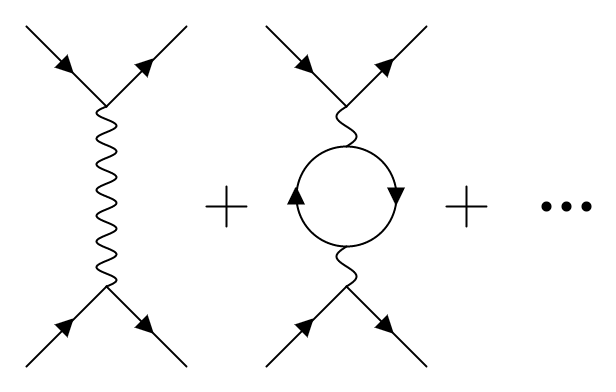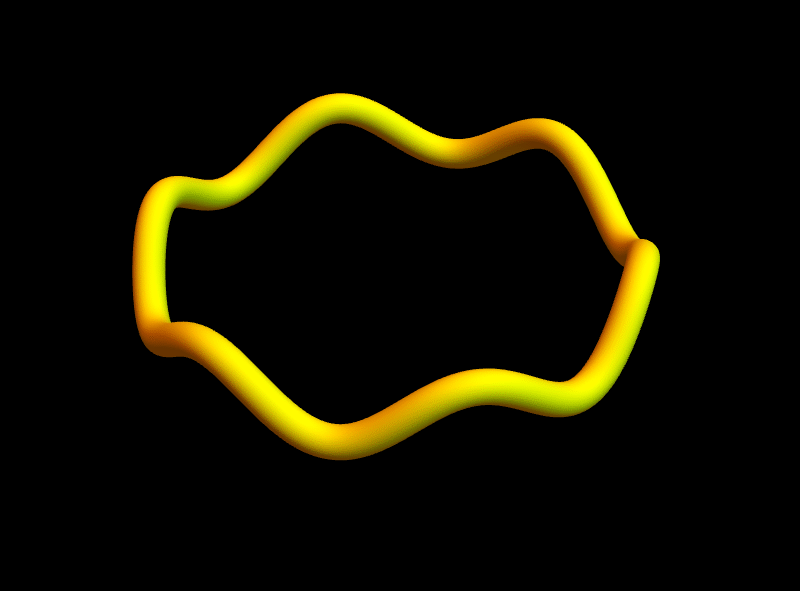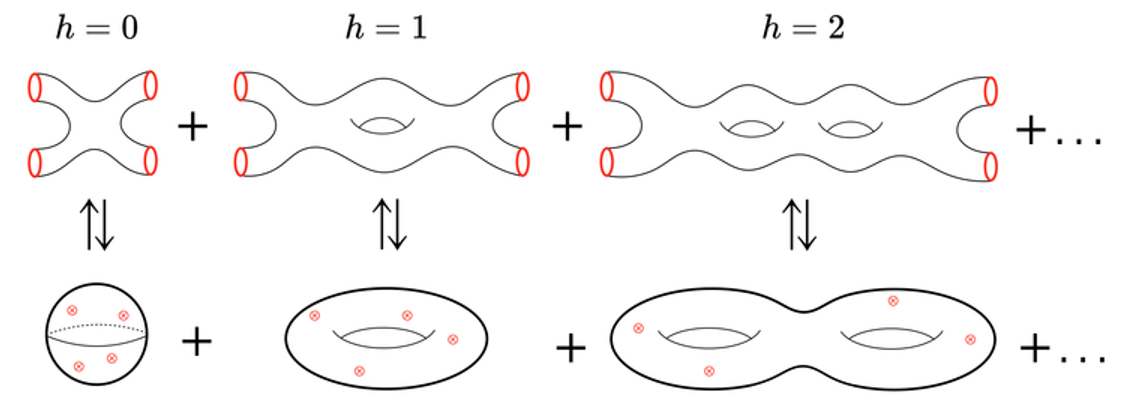# String gravity

Here we are, la partie finale towards getting a grasp on how we can describe the elusive theory of quantum gravity via string theory in light of Feynman diagrams. In the first article on the power of Feynmans‘s doodles we learned that, in order to describe quantum-mechanical phenomena like the scattering of two electrons, we need to include an infinite amount of Feynman diagrams. In that case we would describe the interaction between the electrons via a force-carrying particle, namely the photon. In the second article, Geometric Gravity, we discovered that the force of gravity was a consequence of the curvature of space and time itself.

Now we want to combine both ideas and see how the interactions of strings lead to a quantum theory of gravity.

## Strings vs particles

So far we have encountered the interaction of two electrons, which repel each other by exchanging a photon, which by itself could do all sorts of things. To express the full scattering process one needs to take into account all possible things that can happen. In order to describe these interactions we made use of Feynman diagrams.Figure 2. Ordinary Feynman diagrams. Represented is the interaction of electrons (lines with arrows) exchanging a single photon (wavy line). The additional diagrams correspond to quantum corrections.

Regarding the problem of quantum gravity, however, pointlike particles, like electrons and photons, do not have the properties that physicists believe the quanta of gravity, namely the force-carrying gravitons, should have.

This brings us to string theory, where, instead of pointlike particles, we consider all fundamental particles to be extended objects, namely the infamous strings. Like a guitar string, these strings can also be plucked leading to different vibrations. Amazingly, if the string forms a closed loop one finds that the way the string vibrates can reproduce the properties of the elusive graviton!Figure 3. Vibrating closed string. The vibrational modes of the string have the same properties as gravitons are expected to have. Source: Strings and Holography by Marcel Vonk.

Additionally, we can study the quantum collisions of these strings, as we did for the electrons, and find their ‘stringy’ Feynman diagrams:

But how is this related to a theory for quantum gravity?

## Quantum Gravity

The exceptional fact is that these diagrams illustrate the quantum interactions of strings, which can be linked to the curvature of spacetime and hence gravity.Figure 5. Stringy diagrams and their topology. Recall that different diagrams for the electron scattering were labelled by the number of times the electric charge appears. Here the similar label h counts the number of holes of the stringy diagram. Image: Etienne Dreyer.

In the ordinary, non-stringy case we saw that the additional diagrams described the fluctuations of the electromagnetic field (the photon field). Here we see that the interaction of strings leads to additional stringy diagrams, which are labelled by the number of holes analogously to the way the power of the electric charge appeared in the ordinary Feynman diagrams.

When looking at the first diagram (labelled by $$h = 0$$) we see that, as the two strings interact, they merge and excite a graviton, which then decays into two strings again. Importantly, as strings are thought to be the fundamental constituents of all particles, we see that all particles interact through a universal force: the known gravitational force. From our understanding of ordinary Feynman diagrams we can then observe that the additional stringy Feynman diagrams describe the fluctuations of the gravitational field (the graviton) and therefore describe the quantum-mechanical behaviour of gravity.

Et voilà, by looking at the stringy Feynman diagrams of interacting closed strings, we arrived at a quantum theory for gravity! String theory allows us to describe the quantum-mechanical behaviour of what Einstein taught us more than a century ago. But there are still some mysteries regarding strings and gravity. For those who are intrigued about how this story continues I refer you to Marcel Vonk’s Strings and Holography series.